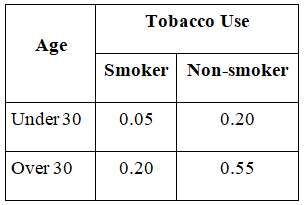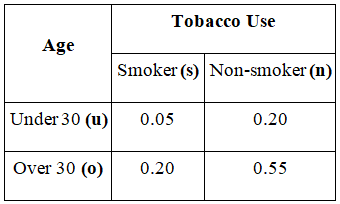# MA 121 : Introduction to Statistics coaching session 2.1.1 #21

21) Hi this is Asmita! I am a Student Success Coach and will be explaining some practice problems from the MA121 Introduction to Statistics course. Today we will review an assigned problem from Unit 2. In the “Homework Assessment” you were asked to solve this problem:

The following two-way contingency table gives the breakdown of the population in a particular locale according to age and tobacco usage:A person is selected at random. Find the probability of each of the following events.
a.The person is a smoker.
b.The person is under 30.
c.The person is a smoker who is under 30.

The first step in approaching this problem is to write the sample space for the experiment and identify the probabilities for each outcome.

The experiment is the action of selecting a person from the population of a particular locale. Label each outcome as a pair of letters, first of which represents age
(u: under 30, o: over 30) and second represents tobacco use (s: smoker, n: non-smoker).With all possible outcomes, sample space can be written as S = {us,un,os,on}
From the given table,

Probability of a person whose age is under 30 and who is a smoker is P (us)= 0.05.

Probability of a person whose age is under 30 and who is a non-smoker is
P (un)= 0.20

Probability of a person whose age is over 30 and who is a smoker is P (os)= 0.20

Probability of a person whose age is over 30 and who is a non-smoker is
P (on) = 0.55

The concept that needs to be used is definition of “Probability of event.”
Steps to find probabilities are as follows:

1. Define the event.
2. Write all possible outcomes for each event.
3. Use the definition of “Probability of an event.”
4. Plug the values to find the required probability.

a.
Step 1: Define the event:
Event T is the action of selecting the person who is a smoker.
This means a person will be smoker and can either be of age under 30 or over 30.

Step 2: Write all possible outcomes in event T:
Outcomes us and os from sample space considers individuals who are smokers (irrespective of age).
Therefore, T = {us ,os}

Step 3: Use definition of “Probability of an event”:
To find the probability of the event, we find the sum of probability of individual outcomes in the event. Therefore,
P (T) = P (us)+ P (os)

Step 4: Plug the values to find required probability:
P (T) = P (us)+ P (os) = 0.05 +0.20 = 0.25

b.
Step 1: Define the event:
Event U is the action of selecting a person who is under 30.
This means that the selected person will be under 30 and can either be a smoker or non- smoker.

Step 2: Write all possible outcomes in event U:
Outcomes us and un from sample space considers individuals who are under 30 (irrespective of whether they smoke or don’t).
Therefore, U = {us ,un}

Step 3: Use definition of “Probability of an event”:
To find probability of the event, we find the sum of probability of individual outcomes in the event. Therefore,
P (U) = P (us) + P (un)

Step 4: Plug the values to find the required probability:
P (U) = P (us) + P (un) = 0.05 + 0.20 = 0.25

c.
Step 1: Define the event:
Event US is the action of selecting the person who is a smoker and under 30.
This means the person should be under 30 and is a smoker at the same time.

Step 2: Write all possible outcomes in an event US:
Outcome in sample space, which represents this situation is us.
Therefore, US = {us}

Step 3: Use the definition of “Probability of an event”:
To find the probability of the event, we find the sum of probability of individual outcomes in the event. Therefore,
P (US) = P (us)

Step 4: Plug the values to find the required probability:
As there is only one outcome in event US, P (US) = P (us) = 0.05.

Conclusion:
Probabilities for each of given events are as follows:
a.The person is a smoker = 0.25
b.The person is under 30 = 0.25
c.The person is a smoker who is under 30 = 0.05

In addition to this, I am also attaching the pdf document of the practice problem for your ready reference.

Question 21.pdf (258.3 KB)

Please let me know if you have any question on this problem or on this topic generally. I will be here in the forum for the next hour.